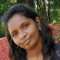Home » Core Java » Java Double Primitive ExampleI have done my Bachelor of Engineering in Computer Science. I have worked primarily in Java and related open source frameworks. Learning and exploring new technologies is my passion

# Java Double Primitive Example

In this article, we will focus on examples in order to understand the Java double primitive type.

## 1. Introduction

The `double` data type is a double-precision 64-bit IEEE 754 floating point. The `double` data type can store fractional numbers from 1.7e−308 to 1.7e+308. That range can also be positive or negative. Note that you should end the value of a double with a “d”.

Example
`double myNum = 34.234d;`

## 2. Double vs Float?

Both double and float data type is used to represent floating point numbers in Java, a double data type is more precise than float.  The precision of a floating point value indicates how many digits the value can have after the decimal point. The precision of `float` is only 6 or 7 decimal digits, while `double` variables have a precision of about 15 or 16 digits. Therefore it is safer to use `double` for most calculations.

## 3. When not to use double?

Let’s look at an example to understand the usage of double for situations involving precision.

DoublePrimitiveExample.java
```package com.javacodegeeks.basic;

public class DoublePrimitiveExample {

public static void main(String[] args) {
double a = 0.1d;
double b = 0.1d;
double c = 0.1d;

double d = a + b + c;
double e = d * 2;
double f = d * 1000;

System.out.println("a + b + c = " + d);
System.out.println("d*2 " + e);
System.out.println("d*1000 " + f);
}

}
```
Output:
```a + b + c = d = 0.30000000000000004
d*2 = 0.6000000000000001
d*1000 = 300.00000000000006
```

In the above example, the problem comes because of how Java stores floating point values in memory. They use the binary representation of the values, and this means that implicitly that values are losing precision. In binary format it is not always possible to store values to the exact precision. So in such cases when currency is involved it is better to use BigDecimal instead of float or double.

## 4. Equality operators on double

Let us consider the following example to understand equality operators on double.

DoubleEqualityExample.java
```package com.javacodegeeks.basic;

public class DoubleEqualityExample {

public static void main(String[] args) {
double x1 = 0.0, y1 = -0.0;
Double a1 = x1, b1 = y1;
System.out.println("x1 == y1 is "+(x1 == y1));       //true
System.out.println("a1.equals(b1) is "+a1.equals(b1));  //false

double x2 = 0.0, y2 = 0.0;
Double a2 = x2, b2 = y2;
System.out.println("(x2 == y2 is "+(x2 == y2));       //true
System.out.println("a2.equals(b2) is "+a2.equals(b2));  //true

double x3 = 0.0/0.0, y3 = 0.0/0.0;
Double a3 = x3, b3 = y3;
System.out.println("x3 != y3 is "+(x3 != y3));       //true
System.out.println("!a3.equals(b3) is "+!a3.equals(b3)); //false
}

}

```
Output:
```x1 == y1 is true
a1.equals(b1) is false
(x2 == y2 is true
a2.equals(b2) is true
x3 != y3 is true
!a3.equals(b3) is false
```

Floating-point equality testing is performed in accordance with the rules of the IEEE 754 standard:

• If either operand is NaN, then the result of `==` is `false` but the result of `!=` is `true`.
• Positive zero and negative zero are considered equal. Ex, `-0.0==0.0` is `true` but -0.0.equals(0.0) is `false` .
• Otherwise, two distinct floating-point values are considered unequal by the equality operators. In particular, there is one value representing positive infinity and one value representing negative infinity, each compares equal only to itself, and each compares unequal to all other values.

This was an example of Java Double Primitive.

You can download the full source code of this example here: Java Double Primitive Example(No Ratings Yet)
Start the discussion Views Tweet it!

# Do you want to know how to develop your skillset to become a Java Rockstar?

## Subscribe to our newsletter to start Rocking right now!

### and many more ....